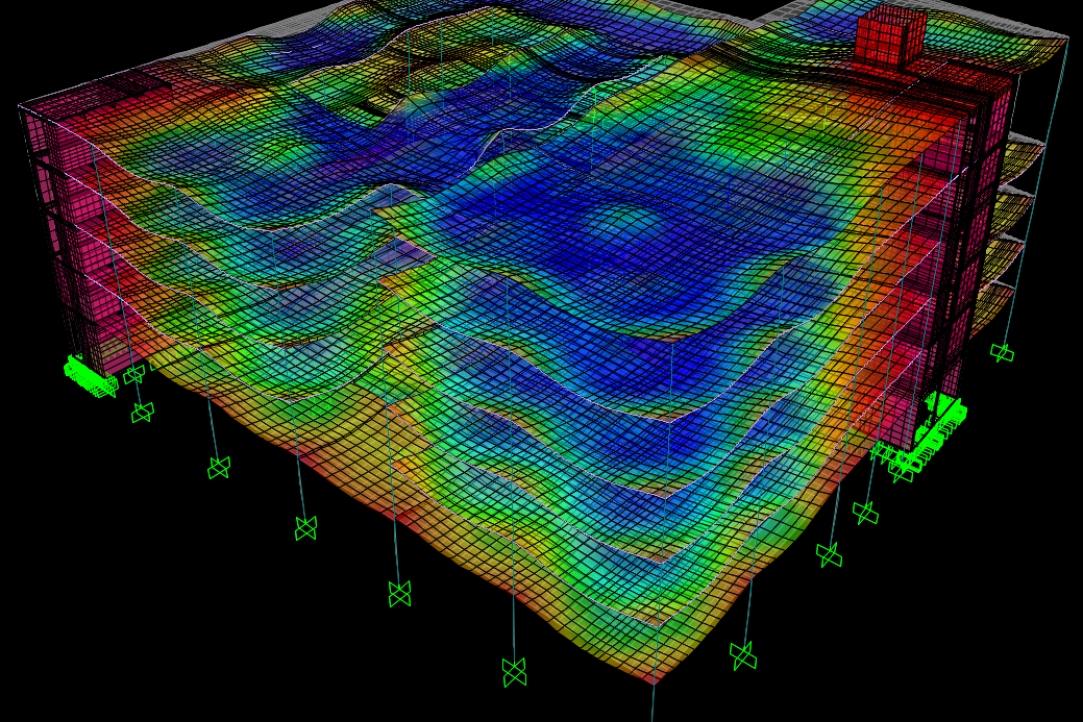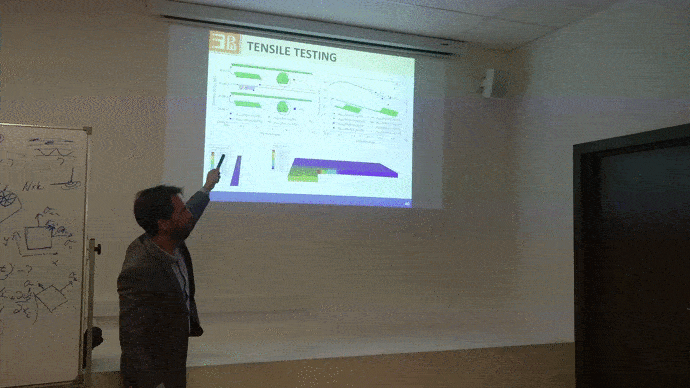• A
• A
• A
• ABC
• ABC
• ABC
• А
• А
• А
• А
• А
Regular version of the site

# On October 19, the Fourth Research Seminar of the Project team was held in a closed format

By the end of the first stage of the Project's work plan implementation, its participants moved from fundamental methodological principles to the applied task of checking and calibrating selected approaches in methodology. These steps can be done only in case of relying on practical development and use of computer simulations. Tat is why some examples of computer simulations use for solving applied problems in the field of material processing by pressure were considered at the seminar. The main speaker was the lead researcher of the Project - Sergei Alekseevich Aksenov.Materials processing by pressure is a field of science that encompasses research on theoretical and technological problems arising mainly in the metallurgical and mechanical engineering industries. Technologies for processing materials by pressure use the property of materials that are amenable to plastic deformation that they change their shape under the influence of external forces and retain it after the end of this influence. Depending on the tasks set, the processes of plastic deformation of materials are investigated by methods of physical, mathematical modeling and computer simulations.

Materials processing computer simulation technologies are used to solve issues of predicting the shape change of solids subjected to complex thermal and mechanical stress. Such problems arise while designing and optimization of technological processes of rolling, forging, stamping, pressing, drawing, sheet forming and their combinations. Technological parameters adjustment is carried out on the basis of the results of computer simulation. That allows to study technological modes in order to minimize the risks of defects formation, optimize the microstructural characteristics of a material, reduce the load on deforming tools, lower operating temperatures, and solve other problems associated with increasing the equipment life and improving product quality.

Finite element modelling is one of the most common methods of computer modelling in the considered subject area. This type of modelling is understood as the construction of simulation models based on boundary value problems in continuum mechanics, the numerical solution of which is carried out by the finite element method. One of the main solid mechanics hypotheses is the continuity hypothesis, which assumes the representation of a real material (consisting of atoms and, therefore, a discrete system) in the form of a simplified model representing the continuum. This idealization allows to formulate a system of partial differential equations, the solution of which is a prediction of the material flow in the considered volume under the given boundary conditions. Since the boundary conditions describing the contact conditions of a solid with deforming tools and the equations of state describing the properties of the material are essentially nonlinear, the analytical solution of the equilibrium equations is not possible in most cases. To construct a solution, a numerical method is used, which assumes the partition of a deformable body volume into elements, within which the displacements of points of the medium are described by functions of a given type (for example, linear ones). This approach makes it possible to pass from a system of partial differential equations to a system of nonlinear algebraic equations, the solution of which, in turn, reduces to a sequence of solving linear systems of the same order. The finite element method is implemented in many commercial CAD systems and is widely used for solving applied problems.

In the report, some specific examples of solving applied problems using finite element modelling were considered. At the same time, special attention was paid to superplastic gas molding processes, which require particularly fine tuning of technological parameters. The effect of superplasticity, which makes it possible to achieve high degrees of deformation without defects, manifests itself in a narrow speed and temperature range. Thus, for each product, it is necessary to calculate the pressure regime so that the strain rates in the workpiece do not leave this range. The process of calculating the pressure regime is automated using finite element modeling.

The key role in materials processing computer modelling is played by the adequacy of the equations specification of state of the material, describing its deformation behaviour. Deformation behaviour is the dependence of material flow stress on deformation, strain rate and temperature. In addition to the fact that information on deformation behaviour is necessary for modelling technological processes, it characterizes the processes occurring in the material that are of interest from the point of view of materials science. Thus, an analysis of the stress-strain curves at a constant temperature and strain rate allows one to judge about the dynamic microstructural processes occurring in the material.A feature of experimental methods for studying the deformation behaviour of materials is that the investigated quantities are not measured directly. The stress value calculation is based on the measured force acting on deforming tool, the strain and strain rate - based on the measured value and the tool travel speed. The calculations are based on various hypotheses that assume uniform deformation of the specimen during the implementation of a particular mechanical test. However, in real experiments, it is impossible to achieve perfect uniform deformation. The report considered the effects of non-uniform distribution of deformations during standard mechanical tests and their contribution to the resulting estimate of deformation behaviour. It was demonstrated that the use of computer modelling methods allows not only to estimate the error of standard methods for studying the deformation behaviour of materials, but also to reduce it through the use of special techniques for processing experimental results.